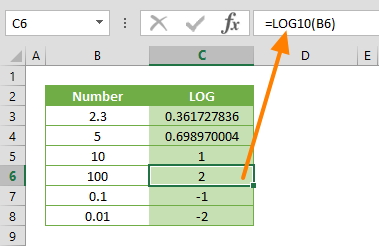The LOG10 Excel function is a Math formula that returns the logarithm of a given number, using base 10. The logarithm of a given number is the exponent to which another fixed number (the base 10) must be raised to produce that number. In this guide, we’re going to show you how to use the LOG10 function in Excel and also go over some tips and error handling methods.

## Supported versions

• All Excel versions

LOG10(number)

## Arguments

 number A positive real number for which you want the logarithm.

## Example

Use the LOG10 Excel function to calculate the logarithm of argument number to the base 10. The equivalent math formula would be log10number. Based on this, we can say that =LOG10(number, 10) is equal LOG10(number). Here is a sample use:

=LOG10(100) returns the logarithm of 100, 2## Tips

• The base is always 10 and the equivalent math formula is =log10number
• Alternatively, you can use LOG function without the [base] argument to use base 10.
• Use the LN function to calculate the natural logarithm of a given number.

## Issues

• The number argument value must be a positive real number. Otherwise, the LOG10 Excel function will return a #NUM! error.